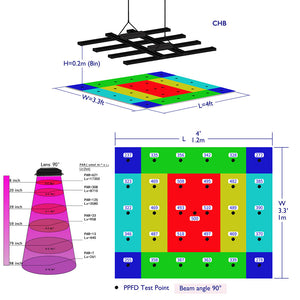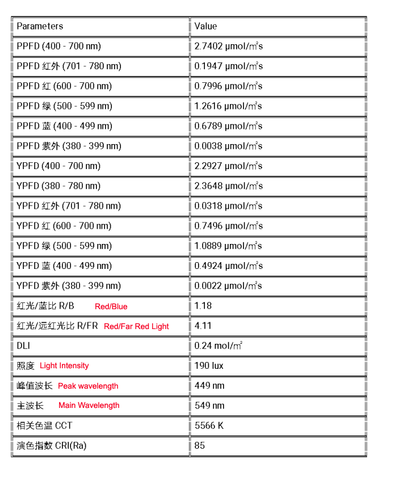PPF, PPFD and YPFD values, What are PAR -

# What are PAR, PPF, PPFD and YPFD values of Plant Growth Lighting ?

The radiation in the specific wavelength range (400-700nm) used by plants for photosynthesis is called photosynthetic effective radiation, and there are two marking units:
One is expressed by photosynthetic irradiance (w / m2), which is mainly used for the generalized study of sunlight photosynthesis.
The second is expressed by photosynthetic photon flux density PPFD (umol / M2S), which is mainly used to study the effects of artificial light source and sunlight on plant photosynthesis.
Using the photon flow radiated to the plant surface per second, this method represents the radiation capacity of the radiation source, which is called PPF_ PAR method.
Par accounts for about 50% of the total solar radiation. It should be noted that the unit of photosynthetic irradiance does not reflect the influence of wavelength.
PPF
Photosynthetic photon flux refers to the number of micro moles of photons radiated by artificial light sources per second in the wavelength band of 400-700nm, in umol / s.
PPFD
Photosynthetic photon flux density and PPF correspond to the number of micro moles radiated by the light source per square meter per second, in umol / M2S, which is the concept of density.
PPFD is the PPF in the range of one square meter.
PPFD is a physical quantity related to the radiation distance, which is inversely proportional to the square of the irradiation distance.
1ppfd represents the number of photons per second radiated on a surface of 1 square meter.
How big is 1umol / M2S (PPFD), which means there are 6 photons in 10 square nanometers.YPF
Generated photon flux
It refers to the number of micro moles of photons radiated per second within the wavelength range emitted by the artificial light source, in umol / s. It is generally considered that the wavelength band is 360-760nm. For LED light source, the wavelength range is set to 380-800nm.
YPFD
Generated photon flux density
Corresponding to YPF is the number of micro moles radiated per square meter per second, in umol / M2S, which is the concept of density.
YPFD is YPF in the range of one square meter.
YPFD is a physical quantity related to the radiation distance, which is inversely proportional to the square of the irradiation distance.
YPFD can more accurately express the light quality and quantity of LED plant lamp.
For red and blue spectra, YPF = PPF, other spectra PPF
The difference between PAR and PPFD is that par is a physical concept, and PPFD is the unit of this physical concept.
Relationship between PPFD and PPF: PPFD is the basic quantity obtained from planting process parameters, and PPF is the main parameter of lamp manufacturing.